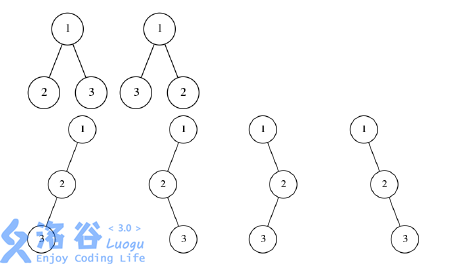P4492 [HAOI2018]苹果树

• 290通过
• 394提交
• 题目提供者 panda_2134
• 评测方式 云端评测
• 标签 割点 树形动规 概率论,统计 各省省选 2018 河南 O2优化
• 难度 NOI/NOI+/CTSC
• 时空限制 1000ms / 512MB
• 提示：收藏到任务计划后，可在首页查看。

题目背景

HAOI2018 Round2 第一题

题目描述

小 C 在自己家的花园里种了一棵苹果树, 树上每个结点都有恰好两个分支. 经过细心的观察, 小 C 发现每一天这棵树都会生长出一个新的结点.

第一天的时候, 果树会长出一个根结点, 以后每一天, 果树会随机选择一个当前树中没有长出过结点 的分支, 然后在这个分支上长出一个新结点, 新结点与分支所属的结点之间连接上一条边.

小 C 定义一棵果树的不便度为树上两两结点之间的距离之和, 两个结点之间 的距离定义为从一个点走到另一个点的路径经过的边数.

现在他非常好奇, 如果 $N$ 天之后小 G 来他家摘苹果, 这个不便度的期望 $E$ 是多少. 但是小 C 讨厌分数, 所以他只想知道 $E \times N !$ 对 $P$ 取模的结果, 可以证明这是一个整数.

输入输出格式

输入格式：

从标准输入中读入数据. 一行两个整数 $N$, $P$ .

输出格式：

输出到标准输出中. 输出一个整数表示答案．

输入输出样例

输入样例#1： 复制
3 610745795
输出样例#1： 复制
24
输入样例#2： 复制
305 1000000007
输出样例#2： 复制
865018107

说明以上是所有 $N = 3$ 时可能的苹果树形态, 其中编号表示这个结点是第几天生 长出来的, 显然每种情况两两结点的距离均为 $4$ .

数据范围与约定

测试点编号 $N$ $P$
$1$ $\le 10$ $\le 10^9 + 7$
$2$ $\le 10$ $\le 10^9 + 7$
$3$ $\le 500$ $\le 10^9 + 7$
$4$ $\le 500$ $\le 10^9 + 7$
$5$ $\le 500$ $\le 10^9 + 7$
$6$ $\le 2000$ $= 10^9 + 7$
$7$ $\le 2000$ $= 10^9 + 7$
$8$ $\le 2000$ $\le 10^9 + 7$
$9$ $\le 2000$ $\le 10^9 + 7$
$10$ $\le 2000$ $\le 10^9 + 7$
提示
标程仅供做题后或实在无思路时参考。
请自觉、自律地使用该功能并请对自己的学习负责。
如果发现恶意抄袭标程，将按照I类违反进行处理。